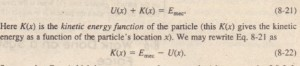# Turning Points

Turning Points

In the absence of a non conservative force, the mechanical energy E of the system has a constant value given byTells us how to determine the kinetic energy K for any location x of the particle: On the U(x) curve, find U for that location x and then subtract. For example. if the particle is at any point to the right . The value  when the particle is at X, and least (0 J)  when the particle is at X.

Note that when the particle reaches XI’ the force on the particle. given  is positive (because the slope  is negative). This means that the particle does not remain at XI but instead begins to move to the right. opposite its earlier motion. Hence XI is a turning point, a place where K = 0 (because U = E) and the particle changes direction. There is no turning point (where K = 0) on the right side of the graph. When the particle heads to the right, it will continue indefinitely.